Changing Text Formatting - WoWInterface
 05-06-17, 08:41 AM #1 cokedrivers A Rage Talon Dragon Guard Join Date: Aug 2009 Posts: 305 Changing Text Formatting As the title says I want to change some text, I have 2 codes but they do not match as things are going on. I would like tyo use the Frist Code for everything. First Code (This code is being used for my Nameplates): Code: ```local FormatValue = function(number) if number < 1e3 then return floor(number) elseif number >= 1e12 then return string.format("%.3fT", number/1e12) elseif number >= 1e9 then return string.format("%.3fB", number/1e9) elseif number >= 1e6 then return string.format("%.2fM", number/1e6) elseif number >= 1e3 then return string.format("%.1fK", number/1e3) end end``` Second Code (This code is being used for my UnitFrames): Code: ``` local shorts = { { 1e10, 1e9, "%.0fB" }, -- 10b+ as 12B { 1e9, 1e9, "%.1fB" }, -- 1b+ as 8.3B { 1e7, 1e6, "%.0fM" }, -- 10m+ as 14M { 1e6, 1e6, "%.1fM" }, -- 1m+ as 7.4M { 1e5, 1e3, "%.0fK" }, -- 100k+ as 840K { 1e3, 1e3, "%.1fK" }, -- 1k+ as 2.5K { 0, 1, "%d" }, -- < 1k as 974 } for i = 1, #shorts do shorts[i] = shorts[i] .. " (%.0f%%)" end hooksecurefunc("TextStatusBar_UpdateTextStringWithValues", function(statusBar, textString, value, valueMin, valueMax) if value == 0 then return textString:SetText("") end local style = GetCVar("statusTextDisplay") if style == "PERCENT" then return textString:SetFormattedText("%.0f%%", value / valueMax * 100) end for i = 1, #shorts do local t = shorts[i] if value >= t then if style == "BOTH" then return textString:SetFormattedText(t, value / t, value / valueMax * 100) else if value < valueMax then for j = 1, #shorts do local v = shorts[j] if valueMax >= v then return textString:SetFormattedText(t .. " / " .. v, value / t, valueMax / v) end end end return textString:SetFormattedText(t, value / t) end end end end)``` Hopefully someone can help me with the code. Thanks Coke Last edited by cokedrivers : 05-06-17 at 09:10 AM.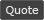05-06-17, 10:09 PM   #2
SDPhantom
A Pyroguard Emberseer
Join Date: Jul 2006
Posts: 1,920
Here's what I've started doing with my number displays.
Lua Code:
`local math_abs=math.abs;local math_floor=math.floor;local math_log10=math.log10;local math_max=math.max;local tostring=tostring; local NumberCaps={"K","M","B","T"};local function AbbreviateNumber(val)    local exp=math_max(0,math_floor(math_log10(math_abs(val))));    if exp<3 then return tostring(math_floor(val)); end     local factor=math_floor(exp/3);    local precision=math_max(0,2-exp%3);    return ((val<0 and "-" or "").."%0."..precision.."f%s"):format(val/1000^factor,NumberCaps[factor] or "e"..(factor*3));end`

It dynamically adjusts the precision based on if the number is in the 1's, 10's, or 100's. It'll revert to scientific notation if the abbreviation accessed is out of the defined scope. You can add more in the NumberCaps table.
__________________Thread Tools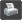Show Printable Version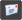Email this Page Display Modes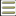Linear Mode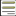Switch to Hybrid Mode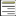Switch to Threaded Mode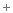Posting Rules You may not post new threads You may not post replies You may not post attachments You may not edit your posts vB code is On Smilies are On [IMG] code is On HTML code is Off